# VBA Variant Data Type (Dim Variable)

## Variant Variable Type

A Variant Variable can hold any time of data (string, integers, decimals, objects, etc.). If you don’t declare a variable type, your variable will be considered variant.

To declare an Variant variable, you use the Dim Statement (short for Dimension):

``Dim varName as Variant``
``Dim rng as Variant``

Then, to assign a value to a variable, simply use the equal sign:

``varName = "John"``
``rng = Sheets(1).Range("A1")``

Putting this in a procedure looks like this:

``````Sub strExample()
'declare the variants
Dim strName As Variant
Dim rng As Variant
'populate the variables
strName = "Fred Smith"
Set rng = Sheets(1).Range("A1")
'populate the sheet
rng.Value = strName
End Sub``````

If you run the code above, cell A1 in Sheet 1 will be populated with “Fred Smith”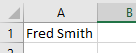By the names declared above, we could conclude that the varName would contain text, and the objSheet would contain a worksheet object.  But actually, any type of data can be directed to the variable.

You could populate the variables declared above as follows and no error would occur.

``varName = 6``
``objSheet - "Fred"``

It is unusual to use variant variables and not considered good practice. On occasions, however, Variant variables are useful.

## Declare Variant Variable at Module or Global Level

In the previous example, you declared the Variant variable within a procedure. Variables declared with a procedure can only be used within that procedure.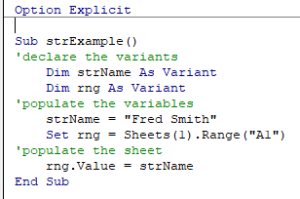Instead, you can declare Variant variables at the module or global level.

### Module Level

Module level variables are declared at the top of code modules with the Dim statement.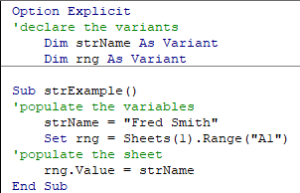These variables can be used with any procedure in that code module.

### Global Level

Global level variables are also declared at the top of code modules. However, instead of using the Dim statement, you use the Public statement to indicate that the string variable is available to be used throughout your VBA Project.

``Public strName as Variant``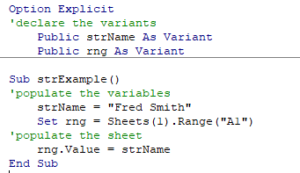If  you declared the variant variable at a module level and used in a different module, an error would occur.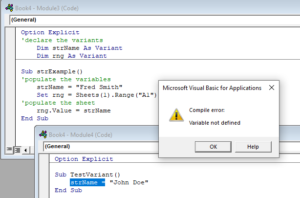If you had used the Public keyword to declare the variant variable, the error would not occur and the procedure would run perfectly.

## Using a Variant to populate Excel

Consider the following procedure:

``````Sub TestVariable
'declare a string to hold the product name
Dim strProduct as string
'declare an integer to hold the product quantity
Dim iQty as integer
'declare doubles to hold the product price, and total price
Dim dblPrice as Double
Dim dblTotal as Double
'populate the variables
strProduct = "All Purpose Flour"
iQty = 3
dblPrice = "\$5.00"
dblTotal = "\$15.00"
'populate the Excel sheet
Range("A1") = strProduct
Range("A2") = iQty
Range("A3") = dblPrice
Range("A4") = dblTotal
End Sub``````

When we run this code, the following error occurs.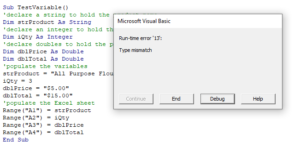Click Debug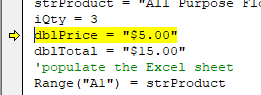You cannot put a dollar sign into the variable as the variable is declared as a Double, and therefore cannot store string values.

Declare dblPrice and dblTotal as Variants which means you are not restricted to a data type.

``````Dim dblPrice as Variant
``````
``Dim dblTotal as Variant``

Re-run the code and the data will appear in the Excel sheet as it should.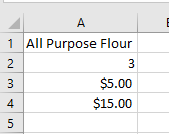Note that the data entered in A4 and A5 are automatically then converted by Excel to numbers.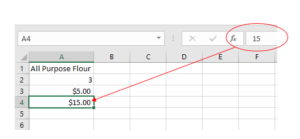## Declaring a Dynamic Array

Variant variables are also useful when you are declaring a dynamic array as they allow the size of the array to change during run-time.

With a Variant Array, you do not need to define the array size. The size will automatically adjust.

``````Sub VariantArray()
Dim arrList() As Variant
'Define Values
arrList= Array(1, 2, 3, 4)
'Change Values
arrList= Array(1,2,3,4,5,6)
'Output Position 4
MsgBox arrVar(4)
End Sub
``````

Stop searching for VBA code online. Learn more about AutoMacro - A VBA Code Builder that allows beginners to code procedures from scratch with minimal coding knowledge and with many time-saving features for all users!